• 主要介绍了Java四舍五入时保留指定小数位数的五种方式，帮助大家更好的理解和使用Java，感兴趣的朋友可以了解下
• ## java保留两位小数

千次阅读 2019-08-09 11:32:03
java保留位小数 1.使用java类库中自带的DecimalFormat类，使数字输出结果保留2位小数 代码如下: 运行结果如下: 2. 输出结果为: String.format表示字符串的格式化 3.使用BigDecimal四舍五入方法 需要导入...
java保留两位小数 1.使用java类库中自带的DecimalFormat类，使数字输出结果保留2位小数 代码如下: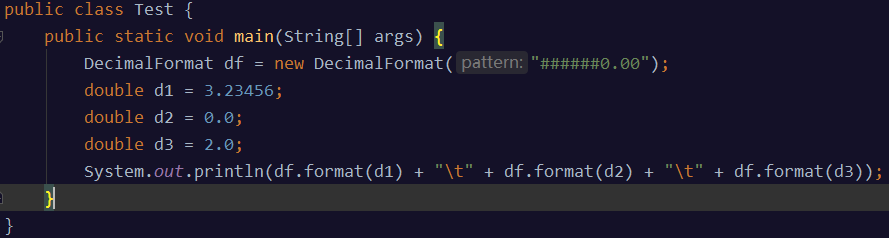运行结果如下: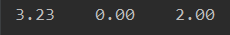2.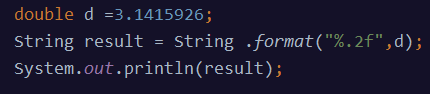输出结果为: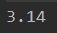String.format表示字符串的格式化
3.使用BigDecimal四舍五入方法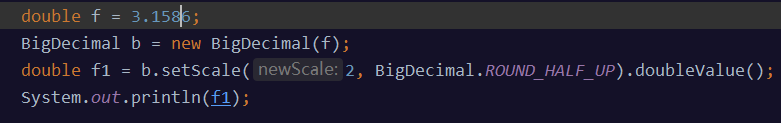需要导入BigDecimal类:import java.math.BigDecimal; 输出结果为: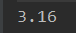1：scale指的是你小数点后的位数。比如123.456则score就是3. score()就是BigDecimal类中的方法啊。 比如:BigDecimal b = new BigDecimal(“123.456”); b.scale(),返回的就是3. 2：roundingMode是小数的保留模式。它们都是BigDecimal中的常量字段,有很多种。 比如：BigDecimal.ROUND_HALF_UP表示的就是4舍5入。 setScale(1)表示保留一位小数，默认用四舍五入方式 setScale(1,BigDecimal.ROUND_DOWN)直接删除多余的小数位，如2.35会变成2.3 setScale(1,BigDecimal.ROUND_UP)进位处理，2.35变成2.4 setScale(1,BigDecimal.ROUND_HALF_UP)四舍五入，2.35变成2.4 setScaler(1,BigDecimal.ROUND_HALF_DOWN)四舍五入，2.35变成2.3，如果是5则向下舍
原文链接：https://blog.csdn.net/ochangwen/article/details/51531866
展开全文• 主要介绍了Java中Double保留小数位的几种方法，文中通过示例代码介绍的非常详细，对大家的学习或者工作具有一定的参考学习价值，需要的朋友们下面随着小编来一起学习学习吧
• 给定两个整数相除，要求输出结果保留一位小数，且计算结果一位小数位数直接保留，其他位直接舍去。 比如，计算结果为： 2.89 则应输出2.8 2.84 则应输出2.8 也就是不按照四舍五入的标准输出。 代码如下： int a; int...
给定两个整数相除，要求输出结果保留一位小数，且计算结果一位小数位数直接保留，其他位直接舍去。 比如，计算结果为： 2.89 则应输出2.8 2.84 则应输出2.8 也就是不按照四舍五入的标准输出。 代码如下：
int a;
int b;
//将计算结果转化为double类型的，只需乘以1.0即可
double result = a*1.0/b;
//减去0.05后按照四舍五入的标准输出
System.out.println(String.format("%.1f",result-0.05));

总结：保留一位小数的话，减去0.05；保留两位小数的话，减去0.005；依次类推，就可以使结果是不按四舍五入的结果。
另一种方法按照四舍五入的方法保留一位小数如下：
int a;
int b;
double result = a*1.0/b;
DecimalFormat f = new DecimalFormat("#0.0");
String d = f.format(result);
System.out.println(d);

展开全文• UI的需求就是返回给前端的数据要保留一位小数： 后端的类型是double类型： double rateA=0; double rateB=0; double rateC=0; double rateD=0; 经过计算后的结果保留一位小数，我们用String.format("%.1f...
UI的需求就是返回给前端的数据要保留一位小数：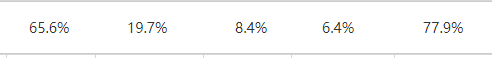后端的类型是double类型：
   double rateA=0;
double rateB=0;
double rateC=0;
double rateD=0;
经过计算后的结果保留一位小数，我们用String.format("%.1f",rateA)来实现就可以了：
 String.format("%.1f",rateA);
String.format("%.1f",rateB);
String.format("%.1f",rateC);
String.format("%.1f",rateD);
输出结果：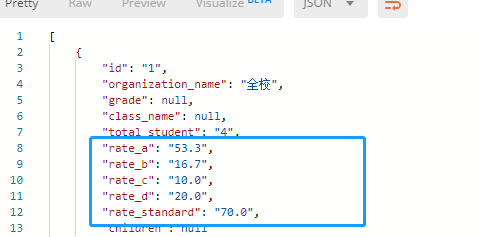展开全文• 文章目录、通过 BigDecimal 类实现二、通过String 实现 、通过 BigDecimal 类实现 主要是通过 BigDecimal 类中的setScale 方法，或者转换为...其中 setScale 方法需要传入两个参数，个是要保留的位数 newSca...


文章目录
一、通过 BigDecimal 类实现二、通过String 实现

一、通过 BigDecimal 类实现
主要是通过 BigDecimal 类中的setScale 方法，或者转换为字符串
源码：
public BigDecimal setScale(int newScale,int roundingMode)

其中 setScale 方法需要传入两个参数，一个是要保留的位数 newScale，另一个是要保留的类型。
BigDecimal.ROUND_HALF_UP 和 BigDecimal.ROUND_HALF_DOWN 都表示四舍五入
import java.math.BigDecimal;

public class Test{
public static void main(String[] args) {
double date = 123.456;
double d1,d2;
BigDecimal b = new BigDecimal(date);
d1 = b.setScale(2,BigDecimal.ROUND_HALF_UP).doubleValue();
d2 = b.setScale(2,BigDecimal.ROUND_HALF_DOWN).doubleValue();
System.out.println(d1);
System.out.println(d2);
}
}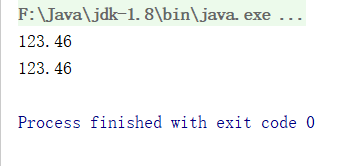2. ROUND_UP，只要弃位非0就进一位
import java.math.BigDecimal;

public class Test{
public static void main(String[] args) {
double date = 123.436;
double d1,d2;
BigDecimal b = new BigDecimal(date);
d1 = b.setScale(1,BigDecimal.ROUND_UP).doubleValue();
System.out.println(d1);
}
}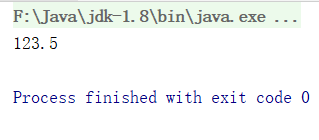3. ROUND_DOWN，不管如何都不会进位
import java.math.BigDecimal;

public class Test{
public static void main(String[] args) {
double date = 123.466;
double d1,d2;
BigDecimal b = new BigDecimal(date);
d1 = b.setScale(1,BigDecimal.ROUND_DOWN).doubleValue();
System.out.println(d1);
}
}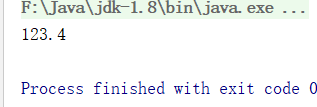4. ROUND_CEILING，向正无穷方向舍入
若为正数，类似于ROUND_UP
若为负数，类似于ROUND_DOWN

import java.math.BigDecimal;

public class Test{
public static void main(String[] args) {
double date1 = 123.466777;
double date2 = -123.466777;
double d1,d2;
BigDecimal b1 = new BigDecimal(date1);
BigDecimal b2 = new BigDecimal(date2);
d1 = b1.setScale(2,BigDecimal.ROUND_CEILING).doubleValue();
d2 = b2.setScale(2,BigDecimal.ROUND_CEILING).doubleValue();
System.out.println(d1);
System.out.println(d2);
}
}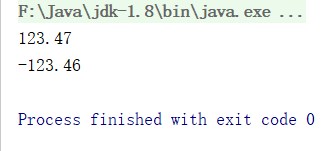5. ROUND_FLOOR，向负无穷方向舍入
java
若为负数，类似于ROUND_UP
若为正数，类似于ROUND_DOWN

import java.math.BigDecimal;

public class Test{
public static void main(String[] args) {
double date1 = 123.466777;
double date2 = -123.466777;
double d1,d2;
BigDecimal b1 = new BigDecimal(date1);
BigDecimal b2 = new BigDecimal(date2);
d1 = b1.setScale(2,BigDecimal.ROUND_FLOOR).doubleValue();
d2 = b2.setScale(2,BigDecimal.ROUND_FLOOR).doubleValue();
System.out.println(d1);
System.out.println(d2);
}
}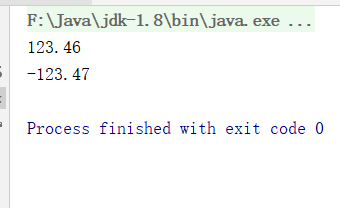二、通过String 实现
%.2f 表示保留后两位，能四舍五入
public class Root{
public static void main(String[] args) {
double d = 123.456;
String result;
result = String.format("%.2f",d);
System.out.println(result);
}
}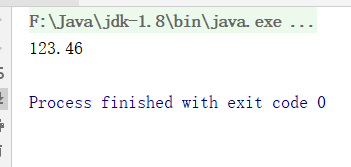`
展开全文• 首先让我们来定义三个变量 double x = 5.112;... 最后一个用于演示一位小数的情况 方法一：使用String.format方法(四舍五入) System.out.println(String.format("%.2f", x)); System.out.println(S...format
• java 中常见的保留位小数的操作，通常都会用到BigDecimal 类 例如： /** * 四舍五入 */ @Test public void test() { double num = 111231.5585; BigDecimal b = new BigDecimal(num); //保留2位小数 ...字符串
• Java四舍五入保留1位小数的方法： import java.math.BigDecimal; public class Test { public static void main(String[] args) { double d1 = 3.02; System.out.println(d1); //利用字符串格式化的...四舍五入
• 不是因为题目有多难，主要卡在了题目上要求保留6位小数，这就非常尴尬了，结果都做对了，就是不会保留，导致题目的通过率为0。在考试结束后就立马查了一些资料，发现了几种保留小数位的方法，都很实用，总结如下： ...
• //保留2位小数 double f =new BigDecimal((float)1/3).setScale(2,BigDecimal.ROUND_HALF_UP).doubleValue(); //百分号处理 int f1 = new BigDecimal((float) jigeSum / n * 100).setScale(0, BigDecimal.ROUND_HALF...算法
• import java.util.*; public class Main{ public static void main(String[] args){ Scanner scanner = new Scanner(System.in); double n = 1.199; System.out.printf("%.2f\n",n);//四舍五入 ...
• “月落乌啼霜满天,江枫渔火对愁眠”很多时候,我们在用java写程序时,要展示一些数据,比如同比,占比,环比之类的,这个时候就难免会遇到小数,一般都是保留位小数.但是会出现这种情况,保留位小数,如果整数位是零的话,...
• Java 保留位小数位 推荐写法： java.text.DecimalFormat df =new java.text.DecimalFormat("#.###");  df.format(0.123); 特别纠正网络部分回答的错误说法： 错误写法如下： double d = 0.0211;  Syst
• //整数相除 保留一位小数 public static String division(int a ,int b){ String result = ""; float num =(float)a/b; DecimalFormat df = new DecimalFormat("0.0"); result = df.format(n
• 、使用BigDecimal，保留小数点后两 public static String format1(double value) { BigDecimal bd = new BigDecimal(value); bd = bd.setScale(2, RoundingMode.HALF_UP); return bd.toString(); } 二、...
• java 两个整数相除保留位小数： https://blog.csdn.net/qq_26676207/article/details/53375263 1、方法1 int a=1099; int b=93; double f1 = new BigDecimal((float)a/b).setScale(2, BigDecimal.ROUND_HALF_...
• ## java保留n位小数输出的几种方法

万次阅读 多人点赞 2019-06-19 22:19:58
、四舍五入吧并保留位小数 类似于c语言printf的输出 printf（）： double x = 8.055; System.out.printf("%.2f\n",x);//8.06 format（）： double x = 8.055; System.out.format("%.2f\n",x);/...
• java保留一位小数，并且四舍五入的实现方法 /** * 方式一： *获取一位四舍五入的小数 */ public static Double getNumber(Double num){ BigDecimal finalNum = new BigDecimal(num).setScale(1,BigDecimal....
• //四舍五入 DecimalFormat ...这个进位制度有点扯淡，基本有两种类型，一个就是四舍五入，一个就是小数后的有效数字大于5才进一位，这句话有点不好理解你可以自己运行一下代码试一下，自己就清楚什么意思了。
• 既然能够判断个数值是否为整数，那么就应该有机会将它的整数部分和小数部分分割开来，也就是我们常说的取整（它同时包含了取小数），在我们初学JAVA时，我们常用的方法是强制转换，或者利用BigDecimal.ROUND语句来...
• 项目中有个小需求，两个整数相除，结果需要保留位小数，即1.00、0.50这种数据格式。 以下做法不行，因为两整数相除，小数点以后的数字会被截断，让运算结果为整数类型， int x = 10; int y = 20; int a = x/y...
• double value = Math.floor((double)1.2); BigDecimal b = new BigDecimal(value);  value = b.setScale(2, BigDecimal.ROUND_HALF_UP).doubleValue(); 此时 value = 1.00
• Java保留位小数问题： 方式： 四舍五入  double f = 111231.5585;  BigDecimal b = new BigDecimal(f);  double f1 = b.setScale(2, BigDecimal.ROUND_HALF_UP).doubleValue();  ...Decimal
• ## java保留小数方法

千次阅读 2018-12-26 19:52:11
1.前言 在java的学习中，往往算法有个是属于java的小数点位数保留...@see java保留小数点示例 */ import java.math.BigDecimal; import java.text.DecimalFormat; import java.text.NumberFormat; public class...
• 复制代码代码如下: ...mport java.text.DecimalFormat; DecimalFormat df = new DecimalFormat("######0.00");double d1 = 3.23456double d2 = 0.0;double d3 = 2.0;df.format(d1);df.format(d...
• import java.text.DecimalFormat; DecimalFormat df = new DecimalFormat("#.00"); double d1 = 1.23456 double d2 = 2.0; double d3 = 0.0; system.out.println(df.format(d1),df.format(d2),df.format(d3...

# java保留一位小数java 订阅